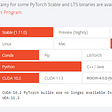# Introduction:

Do you really think that a neural network is a block box? I believe, a neuron inside the human brain may be very complex, but a neuron in a neural network is certainly not that complex.

It does not matter, what software you are developing right now, if you are not getting up to speed on machine learning…you lose. We are going to an era where one software will create another software and perhaps automate itself.

In this article, we are going to discuss how to implement a neural network Machine Learning Algorithm from scratch in Python. This means we are not going to use deep learning libraries like TensorFlow, PyTorch, Keras, etc.

Note that this is one of the posts in the series Machine Learning from Scratch. You may like to read other similar posts like Gradient Descent From Scratch, Linear Regression from Scratch, Logistic Regression from Scratch, Decision Tree from Scratch.

You may like to watch a video version of this article for a more detailed explanation…

# General Terms:

Let us first discuss a few statistical concepts used in this post.

Dot Product of Matrix: Dot product of two matrices is one of the most important operations in deep learning. In mathematics, the dot product is a mathematical operation that takes as input, two equal-length sequences of numbers, and outputs a single number.

Not all matrices are eligible for multiplication. To carry out the dot product of two matrices, The number of columns of the 1st matrix must equal the number of rows of the 2nd. Therefore, If we multiply an m×n matrix by an n×p matrix, then the result is an m×p matrix. Here the first dimension represents rows and the second dimension represents columns in a matrix. Note that the number of columns in the first matrix should be the same as the number of rows in the second matrix. This is represented by the letter n here.

Sigmoid: A sigmoid function is an activation function. For any given input number n, the sigmoid function maps that number to output between 0 and 1.
When the value of n gets larger, the value of the output gets closer to 1 and when n gets smaller, the value of the output gets closer to 0.

Sigmoid Derivative: the derivative of the sigmoid function, is the sigmoid multiplied by one minus the sigmoid.

# Implementation:

## Import Libraries:

We are going to import NumPy and the pandas library.

`import numpy as npimport pandas as pd`

We will be using pandas to load the CSV data to a pandas data frame.

`df = pd.read_csv('Data.csv')df.head()`

To proceed further we need to separate the features and labels.

`x = df[['Glucose','BloodPressure']]y = df['Diabetes']`

After that let us define the sigmoid function.

`def sigmoid(input):        output = 1 / (1 + np.exp(-input))    return output`

There is one more function that we are going to use. It is related to sigmoid and called the sigmoid derivative function.

`# Define the sigmoid derivative functiondef sigmoid_derivative(input):    return sigmoid(input) * (1.0 - sigmoid(input))`

Then we need to define the network training function as below.

`def train_network(features,label,weights,bias,learning_rate,epochs):                                         for epoch in range(epochs):               dot_prod = np.dot(features, weights) + bias        # using sigmoid        preds = sigmoid(dot_prod)        # Error        errors = preds - label                        deriva_cost_funct = errors        deriva_preds = sigmoid_derivative(pred)        deriva_product = deriva_cost_funct * deriva_pred               #update the weights        weights = weights -  np.dot(featurest, deriva_product) * learning_rate                loss = errors.sum()        print(loss)   for i in deriva_product:        bias = bias -  i * learning_rate`

After that let us initialize the required parameters

`np.random.seed(10)features  = xlabel = y.values.reshape(1000,1)weights = np.random.rand(1,2)bias = np.random.rand(1)learning_rate = 0.0004epochs = 100`

We are ready to train the network now:

# End Notes:

In this article, we discussed, how to implement a Neural Network model from scratch without using a deep learning library. However, if you will compare it with the implementations using the libraries, it will give nearly the same result.

The code is uploaded to Github here.

Happy Coding !!

--

--

--

## More from The Startup

Get smarter at building your thing. Follow to join The Startup’s +8 million monthly readers & +756K followers.

## Attention U-Net, ResUnet, & many more## MNIST — Dataset of Handwritten Digits## Some important ML pointers from Andrew NG’s book — ‘Machine Learning Yearning’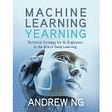## CLASSIFCATION OF FLIGHTS(Airbus/Boeing) USING DIFFERENT ALGORITHMS## Lyft Perception Challenge — 2nd Place Finish## Knowledge Distillation in Neural Networks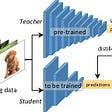## How I Improved A Python Time Series Traffic Problem With Bagging## Dhiraj K

Data Scientist & Machine Learning Evangelist. I like to mess with data. dhiraj10099@gmail.com

## Installing TensorFlow, CUDA, cuDNN with Anaconda for GeForce GTX 1050 Ti## Opening the black box of Machine learning — TensorFlow Dense layers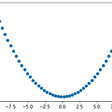## Word Level English to Bengali Machine Translation Using Encoder-Decoder Model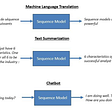## Getting Started PyTorch